# YOLO系列算法综述（2）——YOLO V2算法

### YOLO系列算法综述（2）——YOLO V2算法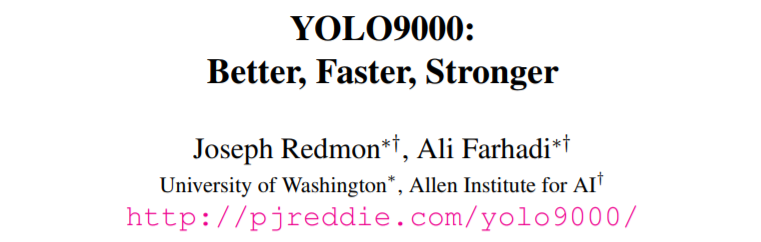# 2 Better（更好）

YOLO V1与最先进的检测系统相比有很多短板，特别是定位错误率高。并且与基于RPN策略的方法相比，YOLO V1的召回率较低。因此，在保证精度的同时，我们将改进的重点放在了提高召回率和定位准确度上。

• Batch Normalization

• High Resolution Classifier

• Convolutional With Anchor Boxes

YOLO V1直接使用卷积特征提取器后的全连接层预测目标的边界框坐标。Faster R-CNN算法使用手动设定的先验框预测边界框坐标，而不是直接进行预测。Faster R-CNN中的RPN网络仅使用卷积层为这些先验框预测置信度和偏移量。由于输出层是卷积层，RPN可以预测特征图中每个位置的偏移量。预测偏移量而不是预测坐标使得问题得以简化，并让网络更容易进行学习。

• Dimension Clusters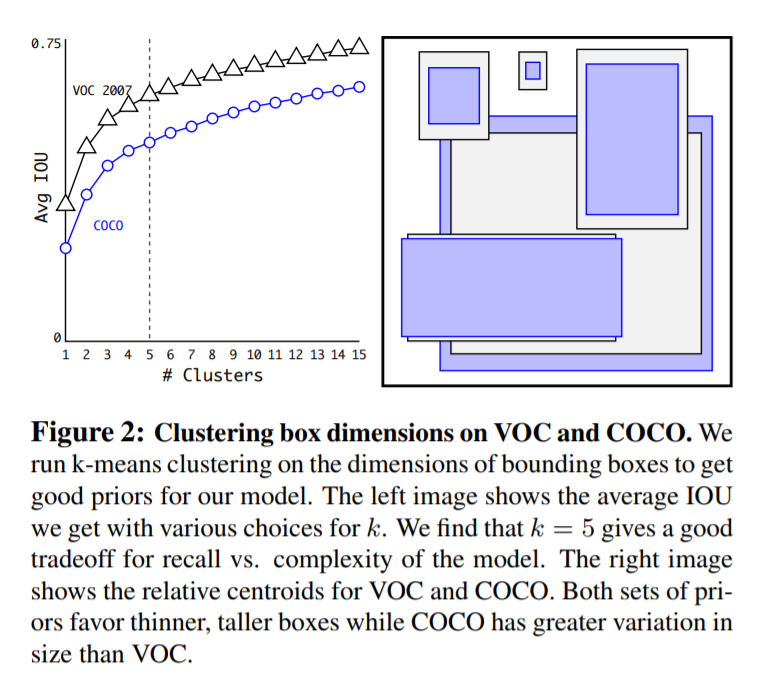d ( b o x , c e n t r o i d ) = 1 − I O U ( b o x , c e n t r o i d ) d(box, centroid) = 1-IOU(box, centroid)

k = 5 k=5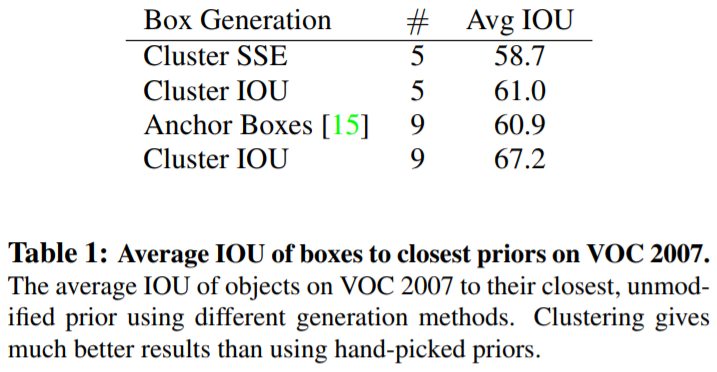• Direct location prediction

x = ( t x ∗ w a ) − x a y = ( t y ∗ h a ) − y a x = (t_x * w_a) -x _a \\ y = (t_y * h_a)-y_a

（ t x ， t y ， t w ， t h ， t o ） （t_x，t_y，t_w，t_h，t_o） 如果单元格是来自图像的左上角的（cx，cy）的偏移，以及前面的边界框有宽度pw和高度ph，那么这些预测符合： b x = σ ( t x ) + c x b y = σ ( t y ) + c y b w = p w ∗ e t w b h = p h ∗ e t h P r ( o b j e c t ) ∗ I O U ( b , o b j e c t ) = σ ( t o ) b_x = \sigma(t_x) + c_x \\ b_y = \sigma(t_y) + c_y \\ b_w = p_w*e^{t_w}\\ b_h = p_h*e^{t_h}\\ Pr(object) *IOU(b,object) = \sigma(t_o) 由于我们限制了位置预测，参数化更容易学习，使网络更稳定。使用尺寸簇以及直接预测边界框中心位置，与使用先验框的版本相比，YOLO提高了近5%。

• Fine-Grained Features

• Multi-Scale Training

YOLO V1使用448x448分辨率的图像作为网络输入，引入先验框时我们将输入改为了416x416。然而，因为我们模型只使用卷积和池层，它可以动态地调整大小。与固定输入大小不同，我们在网络训练中，每10次迭代更换一次网络输入，由于采用了32倍的降采样，因此我们从32的倍数中提取相应分辨率。因此最小的选项是320x320，最大的选项是608x608

• Further Experiments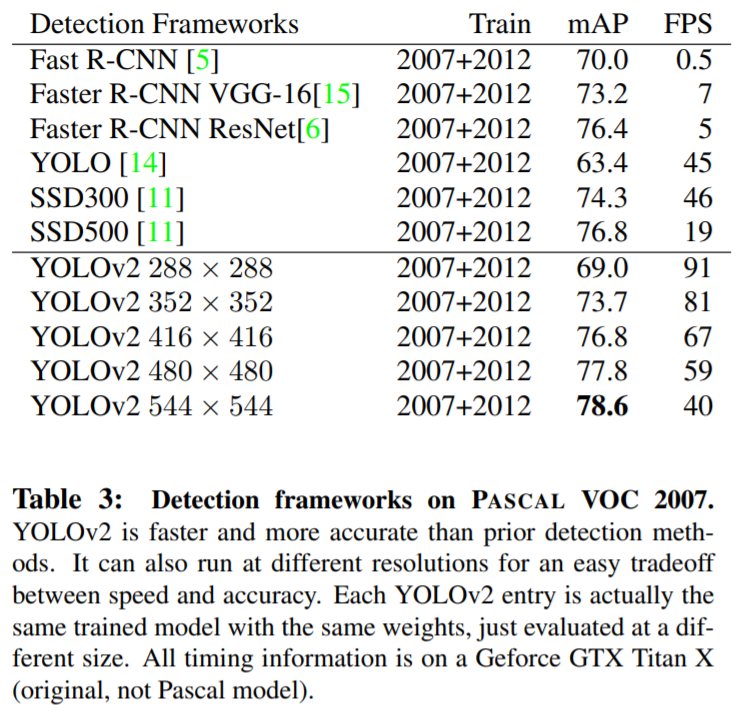# 3 Faster（更快）

YOLO V1和YOLO V2的直观对比：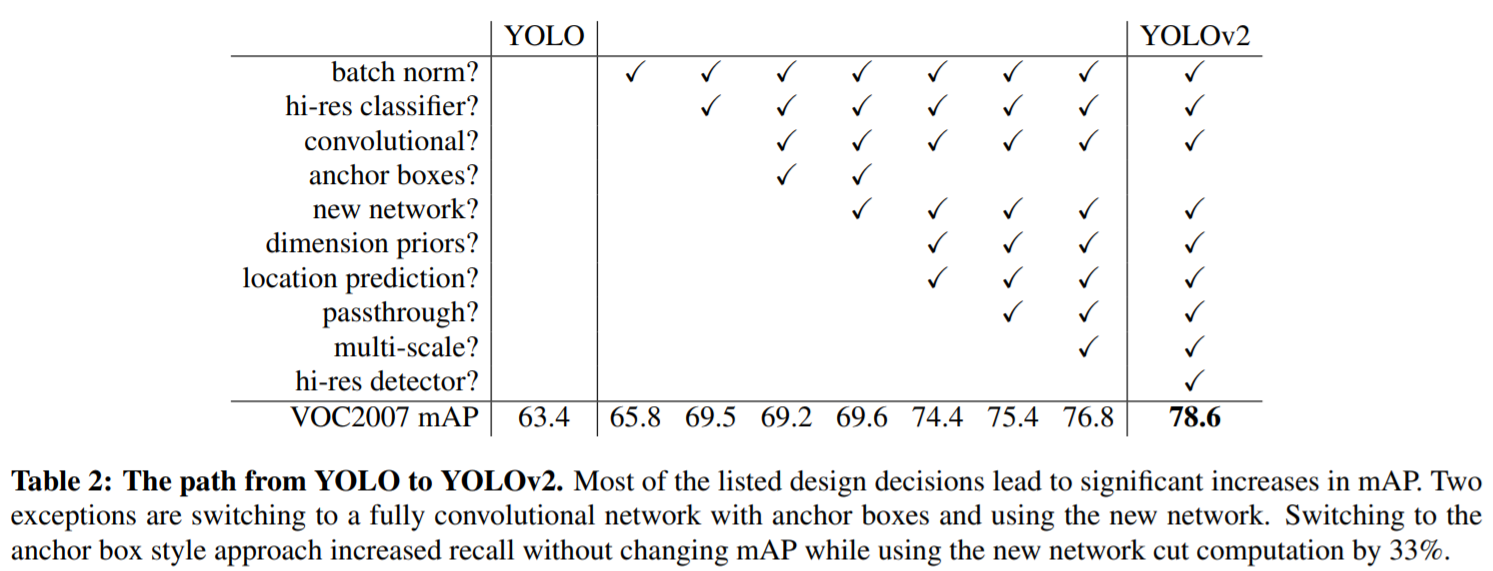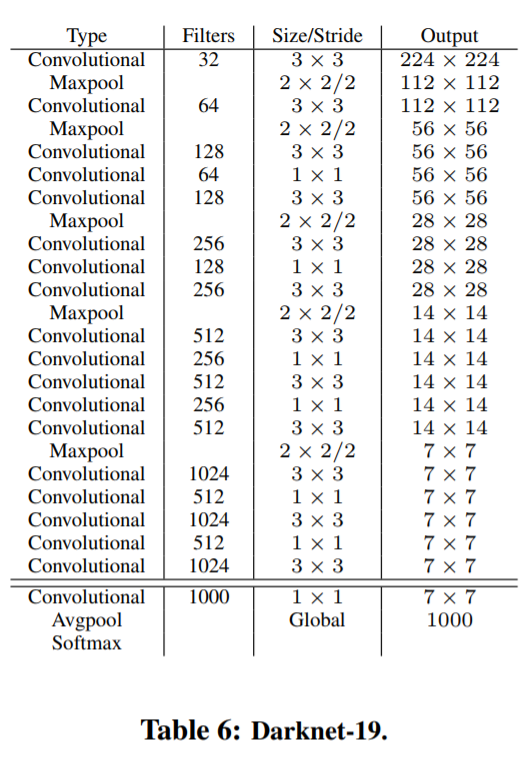# 4 Stronger（更健壮）

terrier“、“Bedlington terrier”等。如果我们想在两个数据集上进行训练，我们需要一种一致的方法来合并这些标签。

• Hierarchical classification

ImageNet标签是从WordNet中提取的，WordNet是一个语言数据库，用于构造概念及其关联方式。在WordNet中，“Norfolk Terrier”和“Yorkshire Terrier”都是“Terrier”的同义词，后者是“狩猎犬”的一种，后者是“狗”的一种，后者是“犬”的一种，等等。大多数分类方法都假定标签为扁平结构，但是为了组合数据集，结构正是我们需要的。

WordNet的结构是有向图，而不是树，因为语言很复杂。例如，“狗”既是“犬”的一种，也是“家畜”的一种，它们都是WordNet中的合成词。我们不使用完整的图结构，而是通过从ImageNet中的概念构建层次树来简化问题。

P r ( N o r f o l k   t e r r i e r ∣ t e r r i e r ) P r ( Y o r k s h i r e   t e r r i e r ∣ t e r r i e r ) P r ( B e d l i n g t o n   t e r r i e r ∣ t e r r i e r ) . . . Pr(Norfolk \ terrier|terrier)\\ Pr(Yorkshire \ terrier|terrier)\\ Pr(Bedlington \ terrier|terrier)\\ ...

P r ( N o r f o l k   t e r r i e r ) = P r ( N o r f o l k   t e r r i e r ∣ t e r r i e r ) ∗ P r ( t e r r i e r ∣ h u n t i n g   d o g ) ∗ . . . ∗ ∗ P r ( m a m m a l ∣ P r ( a n i m a l ) ∗ P r ( a n i m a l ∣ p h y s i c a l   o b j e c t ) Pr(Norfolk \ terrier) = Pr(Norfolk \ terrier|terrier)\\ ∗P r(terrier|hunting \ dog)\\ ∗ . . .∗\\ ∗Pr(mammal|Pr(animal)\\ ∗Pr(animal|physical \ object)

P r ( p h y s i c a l   o b j e c t ) = 1 Pr(physical \ object) = 1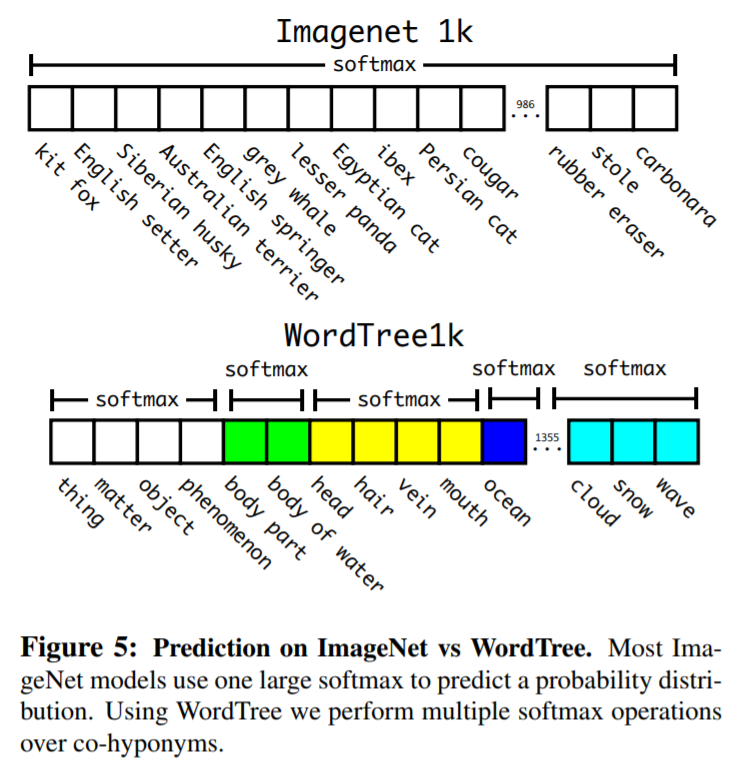• Dataset combination with WordTree

• Joint classification and detection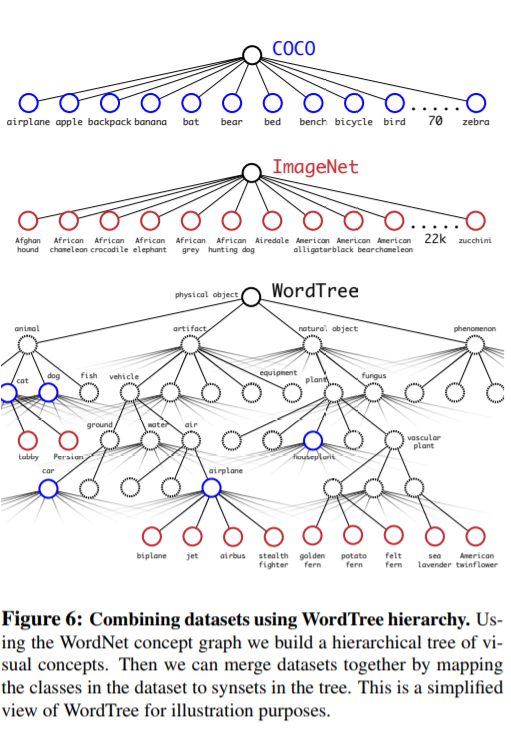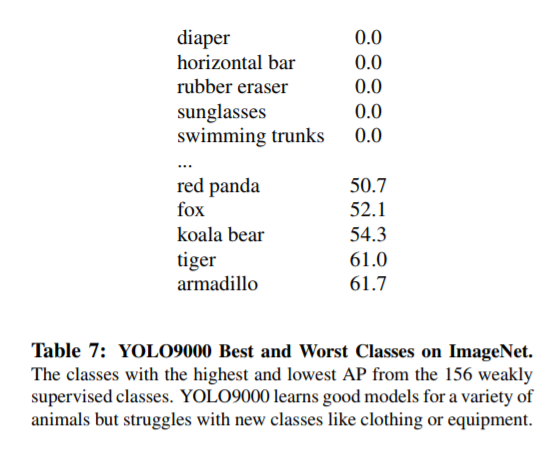# 5 总 结

06-061万+05-26364
03-07923
07-302万+
01-202万+
05-057万+
10-246273
08-18181
08-1590
11-16
05-192081
05-125161
06-05715
07-135229
09-1231万+
10-1815万+
05-231万+
12-062万+
06-042万+
09-134万+